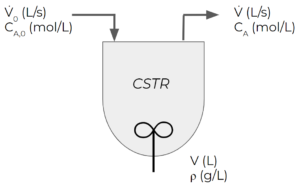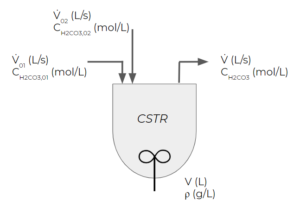# 41 Non-separable Differential Equations

Learning Objectives

By the end of this section, you should be able to:

Provide initial conditions for well-mixed non-separable transient single-unit processes.

Set-up separable transient balances.

## Non-Separable Differential Equations

Non-separable differential equations are differential equations where the variables cannot be isolated. These equations cannot be easily solved and require numerical or analytical methods that will be taught in future courses. You will learn some methods to solve non-separable equations in CHBE 230 (numerical methods) and MATH 256 (differential equations).

$\frac{dy}{dx} = \frac{y}{x} + 1$

You cannot isolate the x and y variables in this differential equation, making it a non-separable ordinary differential equation. Without separation you cannot use the integration techniques you learned in your calculus courses to solve this equation for y as a function of x.

In this class, we will only formulate non-separable differential equations without solving them. We will use general balance equations to come up with differential equations and identify the initial conditions, but we will not find the solutions.

Example: Chemical Reactor

Consider a “continuous stirred-tank reactor” (CSTR). CSTRs are reactors with continuous feed and exit streams and some kind of mixer. Let’s say the following first-order reaction takes place in our CSTR:

$A → B$

Say the units of the rate of consumption of A are $\frac{mol}{s*L}$.

• The feed enters at a volumetric flowrate of $\dot{V}_{0}$ in L/s and a concentration of $C_{A,0}$ in mol/L
• The volume of the tank is constant at $V$ in L and it is perfectly mixed. This means that the concentration of A is uniform within the tank and matches the exit concentration.
• We will assume that the density of all streams in the system is constant at $\rho$ in g/L
• Initially ($t=0$), the tank has an initial concentration of $C_{A,i}$ in mol/L
• The exit stream flows at a rate of $\dot{V}$ in L/s, which is the same as $\dot{V}_{0}$ to ensure our volume stays constantWe want to write a balance for the moles of A in the system under transient conditions.

We start off by writing out the general balance for the moles of A for a CSTR:

$IN-OUT+GEN-CON=ACC$

For this example, we can assume that the reaction is irreversible, indiceted by the one-way arrow in the reaction. Therefore, there will be no $GEN$ term in the mole balance for A. We get the following terms:

$IN = \dot{V}_{0}*C_{A,0}$

$OUT= \dot{V}*C_{A}$

$GEN=0$

$CON= k*C_{A}*V$ – this is the reaction rate in terms of species A

$\frac{dN_{A}}{dt}=\frac{d(V*C_{A})}{dt}=V*\frac{dC_{A}}{dt}$ – since the volume V is constant

The units for all these terms are mol/s. Simplifying the balance, we get:

$\dot{V}_{0}*C_{A,0}-\dot{V}*C_{A}-k*C_{A}*V= V*\frac{dC_{A}}{dt}$

$\frac{\dot{V}_{0}*C_{A,0}}{V}-\frac{\dot{V}*C_{A}}{V}-k*C_{A}= \frac{dC_{A}}{dt}$

with initial conditions: $C_{A}=C_{A,i}$when $t=0$

For this course, formulating this differential equation and listing initial conditions without solving it is sufficient. You will learn how to solve these different equations in future classes.

Exercise: Transient Mole Balance

Consider a CSTR where carbonic acid irreversibly decomposes to form water and carbon dioxide as follows:

$H_{2}CO_{3} → H_{2}O + CO_{2}$There are two streams entering the CSTR that both contain carbonic acid. The first stream has a concentration of $C_{H_{2}CO_{3},01}$ and a volumetric flowrate of $\dot{V}_{01}$, while the second stream has a concentration of $C_{H_{2}CO_{3},02}$ and a volumetric flowrate of $\dot{V}_{02}$. The volume of the reactor is constant V. The concentration of the exit stream is $C_{H_{2}CO_{3},i}=0.5M$ at 0 minutes. If the reaction is first order with the following rate of reaction:

$-r_{H_{2}CO_{3}} = k*C_{H_{2}CO_{3}}$

What is the transient mole balance on carbonic acid? Set up the ordinary differential equation and initial conditions for this system without solving the differential equation.

### Solution

Step 1: Determine what terms are present in the mole balance.

$IN = \dot{V}_{01}*C_{H_{2}CO_{3},01} + \dot{V}_{02}*C_{H_{2}CO_{3},02}$

$OUT= \dot{V}*C_{H_{2}CO_{3}}$

$GEN = 0$

$CON = -r_{H_{2}CO_{3}}*V = k*C_{H_{2}CO_{3}}*V$

$ACC = \frac{dN_{H_{2}CO_{3}}}{dt} = \frac{d(V*C_{H_{2}CO_{3}})}{dt}$

Step 2: Write out the mole balance with the derived terms and determine the initial conditions.

$\dot{V}_{01}*C_{H_{2}CO_{3},01} + \dot{V}_{02}*C_{H_{2}CO_{3},02} - \dot{V}*C_{H_{2}CO_{3}} - k*C_{H_{2}CO_{3}}*V = \frac{d(V*C_{H_{2}CO_{3}})}{dt}$

The intial conditions are $C_{H_{2}CO_{3}}(t=0 min) = 0.5 M$

Note you can see from this equation that $V*C_{H_{2}CO_{3}}$ cannot be isolated. When we try to put all terms containing $C_{H_{2}CO_{3}}$ to the right side by dividing it, it induced $C_{H_{2}CO_{3}}$ terms into the first and second term on the left. and thus we cannot use separation of variables. You will learn to solve these equations in your ordinary differential equations class.